Courses

# RD Sharma Test: Circles

## 25 Questions MCQ Test UPSC Prelims Paper 2 CSAT - Quant, Verbal & Decision Making | RD Sharma Test: Circles

Description
This mock test of RD Sharma Test: Circles for UPSC helps you for every UPSC entrance exam. This contains 25 Multiple Choice Questions for UPSC RD Sharma Test: Circles (mcq) to study with solutions a complete question bank. The solved questions answers in this RD Sharma Test: Circles quiz give you a good mix of easy questions and tough questions. UPSC students definitely take this RD Sharma Test: Circles exercise for a better result in the exam. You can find other RD Sharma Test: Circles extra questions, long questions & short questions for UPSC on EduRev as well by searching above.
QUESTION: 1

### The length of tangent PQ, from an external point P is 24 cm. If the distance of the point P from the centre is 25 cm, then the diameter of the circle is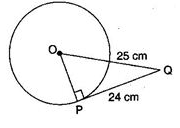Solution:Here ∠OPQ = 90°[Angle between tangent and radius through the point of contact]
∴ OQ2 = OP2 + PQ2 (25)2 = OP2 + (24)2 ⇒ OP2 = 625 - 576 ⇒ OP2 = 49
⇒ OP = 7 cm
Therefore, the diameter = 2 x OP = 2 x 7 = 14 cm

QUESTION: 2

### A tangent PQ at point of contact P to a circle of radius 12 cm meets the line through centre O to a point Q such that OQ = 20 cm, length of tangent PQ is :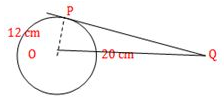Solution:Since op is perpendicular to PQ, the ∠OPQ = 90°
Now, in right angled triangle OPQ,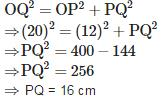QUESTION: 3

### PQ is a tangent drawn from a point P to a circle with centre O and QOR is a diameter of the circle such ∠POR = 120∘, then ∠OPQ is

Solution: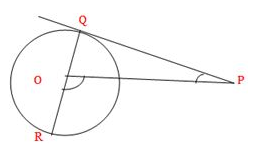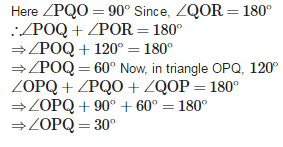QUESTION: 4

A circle is inscribed in ΔABC having sides 8 cm, 10 cm and 12 cm as shown in the figure. Then,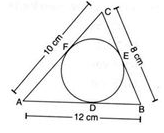Solution:

Let AD = x and BE = y
∴ BD = 12 -  x ⇒  BE = 12 - x [BD = BE = Tangents to a circle from an external point]
⇒ y = 12 - x ⇒ x+y = 12.......(i)
Also, AF = x and CF = 10 - x and CE = 8 - y
Also, AF = x and CF = 10 — x and CE = 8 -  y
∴ 10 - x = 8 - yx - y = 2  (ii)
On solving eq. (i) and (ii), we get x = 7 and y = 5
⇒ AD = 7 cm and BE = 5cm

QUESTION: 5

In the given figure, If TP and TQ are two tangents to a circle with centre O, so that ∠POQ = 110o then ∠PTQ is equal to :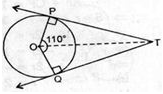Solution:

Since the angle between the two tangents drawn from an external point to a circle in supplementary of the angle between the radii of the circle through the point of contact.
∴ ∠PTQ = 180−110 = 70

QUESTION: 6

A tangent PQ at a point P o a circle of radius 5 cm meets a line through the centre O at point Q, so that OQ = 12 cm. find the length PQ.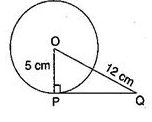Solution:

∠OPQ = 90° [Angle between tangent and radius through the point of contact]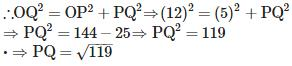QUESTION: 7

From a point Q, the length of tangent to a circle is 24 cm and the distance of Q from the centre is 25 cm, radius of circle is :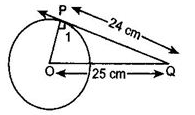Solution:Here ∠OPQ = 90° [Tangent makes right angle with the radius at the point of contact]
∴ OQ2 = OP2 + PQ2 (25)2 = OP2 + (24)2
⇒ OP2 = 676 - 576 = 49
⇒ OP = 7 cm Therefore, the radius of the circle is 7 cm

QUESTION: 8

If PA and PB are tangents to the circle with centre O such that ∠APB = 40, then ∠OAB is equal to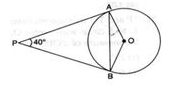Solution:

Let ∠OAB = ∠OBA = x [Opposite angles of opposite equal radii] And ∠AOB =180° -  40° = 140°
Now, in triangle AOB,
∠OAB + ∠OBA + ∠AOB = 180°
⇒ x + x +140° = 180°
⇒ 2x = 40°
⇒ x = 20°
∴ ∠OAB = 20°

QUESTION: 9

Quadrilateral PQRS circumscribes a circle as shown in the figure. The side of the quadrilateral which is equal to PD + QB is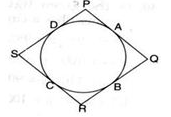Solution:

PD + QB = PA + QA [Tangents from an external point to a circle are equal]
⇒PD + QB = PQ

QUESTION: 10

In the given figure, the pair of tangents A to a circle with centre O are perpendicular to each other and length of each tangent is 5 cm, then the radius of the circle is :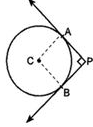Solution:Construction: Joined OP and OQ.
Here OP ⊥ AP and OC⊥AQ and PA ⊥ AQ Also AP = AQ
Therefore. APOQ is a square. ⇒ AP = OP = OQ = 5 cm

QUESTION: 11

In the given figure, RQ is a tangent to the circle with centre O. If SQ = 6 cm and RQ = 4 cm, then OR is equal to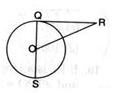Solution:Here SQ = 6 cm, then OQ = OS = 6/2 = 3 cm [Radii] and ∠Q = 90°
Now, in triangle OQR,OR2 = QR2 + OQ2
⇒ OR2 = (4)2 + (3)2 = 16 + 9 = 25
⇒ OR = 5cm

QUESTION: 12

In figure, AB is a chord of a circle and AT is a tangent at A such that ∠BAT = 60o, measure of ∠ACB is :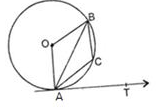Solution:

Since OA is perpendicular to AT. then ∠OAT = 90°
⇒ ∠OAB + ∠BAT = 90°
⇒ ∠OAB + 60° = 90° ⇒ ∠OAB = 30°
∴ ∠OAB = ∠O8A = 30" [Angles opposite to radii]
∴ ∠OAB = 180° - (30° +30° ) = 120° [Angle sum property of a triangle]
∴ Reflex ∠AOB = 360° - 120° = 240°
Now, since the degree measure of an arc of a circle is twice the angle subtended by it any point of the alternate segment of the circle with respect to the arc.
∴ Reflex ∠AOB = 2∠ACB ⇒ 240° = 2∠ACB ⇒ ∠ACB = 120°

QUESTION: 13

If two tangents inclined at 60 are drawn to circle of radius 3 cm, then length of each tangent is equal to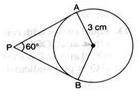Solution:

Let O be the centre. Construction: Joined OP.Since OP bisects ∠P, therefore, ∠APO = ∠OPB = 30° and ∠OAP = 90°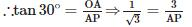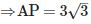cm Since each tangent from an external point to a circle are equal. Therefore, PA = PB = 3√3 cm

QUESTION: 14

The perimeter of ΔPQR in the given figure is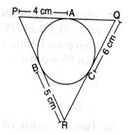Solution:

Since Tangents from an external point to a circle are equal.
∴ PA = PB = 4 cm.
BR = CR = 5 cm CQ = AQ = 6 cm ⇒ Perimeter of ΔPQR = PQ + QR + RP
⇒ Perimeter of ΔPQR = PA + AQ + QC + CR + BR + PB  ⇒ Perimeter of ΔPQR = 4 + 6 + 6 + 5 + 5 + 4
⇒ Perimeter of Δ PQR = 30 cm

QUESTION: 15

In the given fig., if O is the centre of a circle, PQ is a chord and the tangent PR at P makes an angle of 50 with PQ, then ∠POQ is equal to :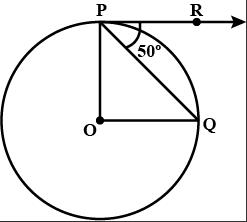Solution:

Since Op is perpendicular to PR, then ∠OPR = 90°
∠RPQ + ∠QPO = 90°
⇒ 50° + ∠QPO = 90°
∠QPO = 40°
Now, OP = OQ (Radii of same circle]
∴ ∠OPQ = ∠OQP = 40°[Angles opposite to equal sides]
In triangle OPQ,
∠POQ + ∠OPQ + ∠OQP = 180°
∠POQ + 40° + 40° = 180°
= ∠ POQ = 100°

QUESTION: 16

In the adjoining figure, the measure Of PR is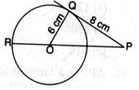Solution:

Here ∠Q = 90° [Angle between tangent and radius through the point of contact] Now, in triangle OPQ, OP2 = QO2 + PQ2
⇒ OP2 = (6)2 + (8)2 = 36 + 64 = 100
⇒ OP = 10 cm
∴ PR = OP + OR = OP + OQ [OR = OQ = Radii]
⇒ PR = 10+6 = 16cm

QUESTION: 17

If tangents PA and PB from a point P to a circle with centre O are inclined to each other at angle of 80o, ∠POA is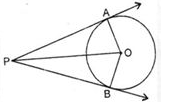Solution: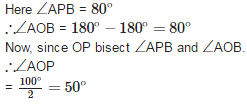QUESTION: 18

If PQR is a tangent to a circle at Q whose centre is O, AB is a chord parallel to PR and ∠BQR = 60, then ∠AQB is equal to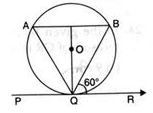Solution:

Since AB II PA and BQ is intersectin.
∴ ∠BQR = LQBA = 60° [Alternate angles]
And ∠BQR = ∠QAB = 60° [Alternate segment theorem] Now, in triangle AQB,
∠AQB + ∠QBA + ∠BAQ = 180°
⇒ ∠AQ,B + 60° + 60° = 180°
⇒ ∠LAQB = 60°

QUESTION: 19

In the given figure, a circle touches all four sides of a quadrilateral PQRS, whose sides are PQ = 6.5 cm, QR = 7.3 cm, and PS = 4.2 cm, then RS is equal to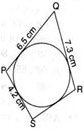Solution:

Let point of contact of RS be A, point of contact of QR be B, point of contact of PQ be C and point of contact of PS be D. Also let AS = x and AR = y
Now, AS = SD = x [Tangents from an external point]

PD = 4.2 - x
But PD = PC= 4.2 - x
And QC = 6.5 - PC = 6.5 - 4.2 + x = 2.3 + x .........(i)
Now, again, AR = BR = y [Tangents from an external point]
⇒ QB = 7.3 -  y
But QB = QC [Tangents from an external point]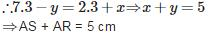QUESTION: 20

In the given figure, AT is a tangent to the circle with centre O such that OT = 4 cm and ∠OTA = 30o. Then AT is equal to :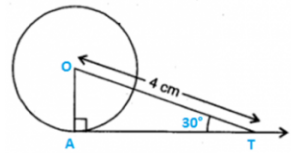Solution:

Construction : Joined OA.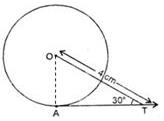Since OA is perpendicular to AT. then ∠OAP = 90°
In right angled triangle OAT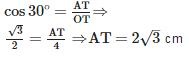QUESTION: 21

In the figure given alongside the length of PR is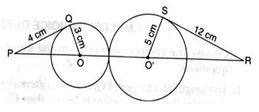Solution:

Here ∠Q = 90° and LS = 90° [Angle between tangent and radius through the point of contact]
Now, in triangle OPQ, OP2 = OQ2 + QP2
⇒ OP2 = (3)2 + (4)2
⇒ OP2 = 16 + 9 = 25
⇒ OP = 5 cm Again in triangle RSO', O'R2 = O'S2 + FS2
⇒ O'R2 = (5)2 + (12)2
⇒ O'R2 = 25 +144 = 169
⇒ O'R = 13 cm
∴ PR = OP+OQ+O's+OR = 5+ 3+ 5+ 13 = 26 m

QUESTION: 22

A tangent PQ at a point P of a circle of radius 5 cm meets a line through the centre O at a point Q, such that OQ = 15 cm. Length of PQ is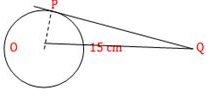Solution:Since OP is perpendicular to PQ, then ∠OPQ = 90°
Now, in triangle OPQ.
OQ2 = OP2 + PQ2 ⇒ (15)2 = (5)2 + PQ2 ⇒ PQ2 = 225 -  25
⇒ PQ2 = 200 ⇒ PQ = 10√2cm

QUESTION: 23

If tangents PA and PB from a point P to a circle with centre O are inclined to each other at an angle of 80, then find ∠POA.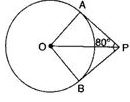Solution:

Here ∠OAP = 90°
And ∠OPA = 1/2 ∠BPA [Centre lies on the bisector of the angle between the two tangents]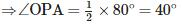Now, in triangle OPA,
∠OAP + ∠OPA + ∠POA = 180°
⇒ 90° + 40° + ∠POA =180°
⇒ ∠POA = 50°

QUESTION: 24

In the given figure, perimeter of quadrilateral ABCD is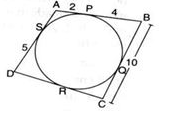Solution:

Here SD = RD = 5 units [Tangents from an external point]
And PB = QB = 4 cm [Tangents from an external point]
∴ QC = 10 - QB = 10 - 4 = 6 units
Also QC = RC = 6 units [Tangents from an extemal point]
∴ CD = RD + RC = 5 + 6 = 11 units
Also AP = AS = 2 units [Tangents from an external point]
∴ AD = AS + DS = 2 + 5 = 7 units Therefore. Perimeter of quadrilateral ABCD = 6 + 10 + 11 + 7 = 34 units

QUESTION: 25

At one end of a diameter PQ of a circle of radius 5 cm, tangent XPYis drawn to the circle. The length of chord AB parallel to XY and at a distance of 8 cm from P is

Solution: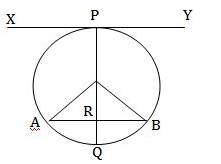Here, OP = 00 = 5 cm [Radii]
And OR = PR -  OP = 8 - 5 = 3 cm Also, OA = 5 cm [Radius]
Now, in triangle AOQ, OA2 = OR2 + AR2 ⇒ 52 = 32 + AR2
⇒AR2 = 25-9= 160 AR= 4cm
Since, perpendicular from centre of a circle to a chord bisects the chord.
∴ AB = AR+ BR = 4+ 4 = 8cm